# Ohm’s Law Vaping Guide

by Jemma Wolfe

By Jemma Wolfe
Published On: 10-05-23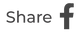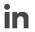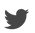• A Guide to Ohms Law For Advanced Vaping
• What is Ohm's Law
• The Forumla for Ohm's Law
• Voltage
• Current
• Resistance
• The Ohm's Law Pyramid
• Ohm's Law Vaping
• Vape Wattage
• Effects of Vape Wattage
• Vape Wattage and Amperage
• Ohm's Law and Advanced Vaping

What is Ohm’s law, and what does it have to do with vaping? If you ever took physics at A-level, it may ring a bell. Whilst we may not instinctively think of scientific laws and equations when we think of vaping, Ohm’s law can be highly useful once you start delving a little deeper into advanced vaping.

However, a lot of this information is useful on just that point: deep diving into advanced vaping. If you’re a beginner whose primary concern is still using vaping to help you quit smoking, you won’t need to think about any of this. Thus, this blog aims to serve as a guide for intermediate to advanced level vape users, and will take you through everything you need to know regarding Ohm’s law in terms of its relationship to vaping. It will also include an examination of vape wattage as it pertains to Ohm’s law and advanced vaping.

We’ll start by breaking down Ohm’s law itself, looking at its expressions and formulations, and how to calculate them. We’ll then use this to examine how and why Ohm’s law matters to vape kits. We understand that working out physics equations may not be the reason you first picked up a vape kit, but — once you get the hang of this simple formula — it can really help you get better stuck into a more advanced, deeper enjoyment of vaping.Ohm’s law is a formula in physical science. It was named after the German physicist Georg Ohm, who in 1827 published a treatise describing measurements of applied voltage and current through simple electric circuits.#### The formula for Ohm’s law

The technical formulation of Ohm’s law is as follows:

“The current through a conductor between two points is directly proportional to the voltage across the two points, provided all physical conditions and temperatures remain constant.”

By introducing to this relationship the constant of proportionality, the resistance, you get a mathematical formula that explains this relationship:

I = V/R

Ohm’s law, then, is most often used in a circuit analysis; circuit analysis simply being a method for calculating current through, and voltage across, electric circuits. This is the same manner in which it is applied when it comes to vaping.

Applying Ohm’s law is fairly straightforward, but in order to do so, let’s examine the three factors in the relationship.#### Voltage

Voltage is the difference in electrical potential between two points. In circuit analysis and when used for Ohm’s law, it corresponds to the work needed to push a charge between the two points. For our purposes, in plainer terms, voltage is what “pushes” electrical current through the circuit.

Voltage is measured in the SI unit volt, and is denoted symbolically as V. For calculations, it is denoted as V.#### Current

Electrical current is a stream of charged particles (e.g. electrons or ions), moving through an electrical conductor. These particles are also called charge carriers. In electric circuits, these charge carriers are typically electrons moving through a wire.

Current is measured in the SI unit ampere (or amp), denoted as the symbol A. For calculations, it is denoted as I.#### Resistance

Electrical resistance is a measure of a given object’s opposition to the flow of electrical current — that is, its resistance to current. The higher the resistance of a given electric circuit, the lower its current. (Conceptually, electrical resistance is similar to mechanical friction.)

Resistance is measured in the SI unit ohm, denoted as the symbol . For calculations it is denoted as R.

Now that we have an understanding of the three variables that form Ohm’s law, we can look at how to calculate them.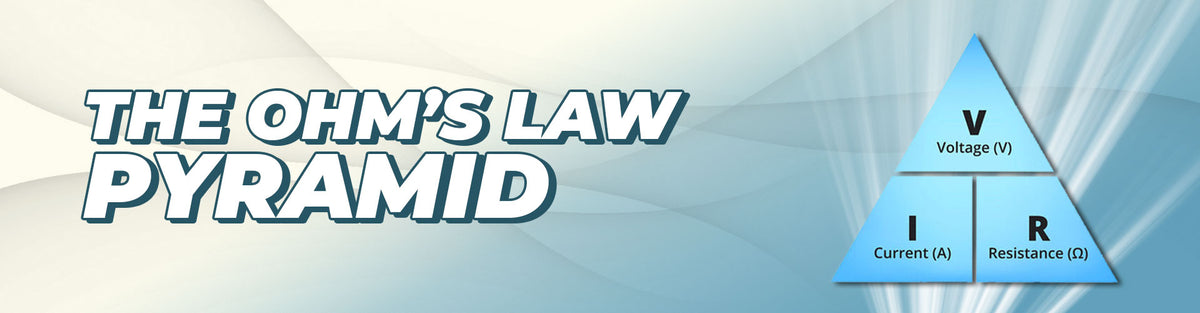#### The Ohm’s law “pyramid”

In circuit analysis, there are three expressions of Ohm’s law; they are equivalent, and are used interchangeably. The three expressions are as follows:

I = V/R or V = IR or R = V/I .

These three expressions allow you to calculate an unknown variable, based on the other two variables. Thus,

● To solve for current (I), divide voltage (V) by resistance (R).
● To solve for voltage (V), multiply current (I) by resistance (R).
● To solve for resistance (R), divide voltage (V) by current (I).

The expressions for Ohm’s law are often represented visually as this pyramid. In this image mnemonic, covering the unknown variable will give the remaining parameters. For example, covering I will give V/R.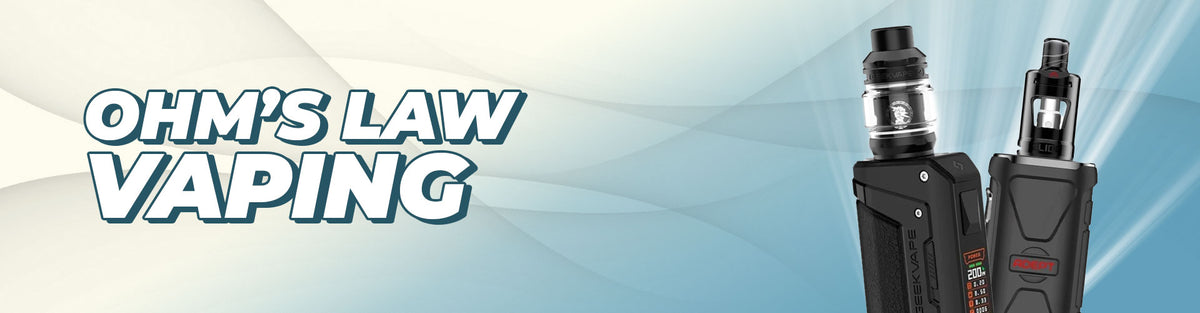### Ohm’s Law Vaping

So now that we know how to calculate Ohm’s law, we can ask: what’s its relevance for your vape kit? Although we’ve explained the three equivalent expressions for Ohm’s law, there’s a case to be made that one expression in particular takes precedence:

I = V/R .

Why is solving for current the most important to remember? The main reason is that, in the electric circuit that is a vape kit, V and R are both fixed values.

● Voltage is supplied by the vape battery, and is fixed. For example, the 18560 Lithium-ion battery (the industry standard external battery for advanced vape kits) has a nominal voltage of 3.6V.

● Resistance comes from the vape coil. Every vape coil has a fixed value resistance in ohms. Obviously, you can change the resistance of your overall vape kit setup by switching coils, but you can’t change the value of each coil itself — thus, when calculating, you would consider it a fixed value based on the currently installed coil.

What this means is that normally — in real-word circumstances — you will already know the value of V and R, so you would simply use those two to solve for I.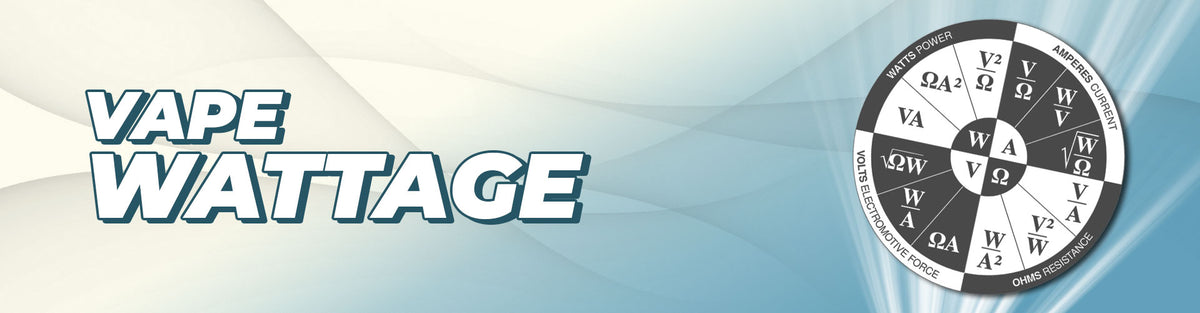### Vape Wattage

Another factor we haven’t examined yet is wattage, or more simply electric power. This is the rate at which electrical energy is transferred by an electric circuit.

The SI unit of power is the watt, denoted symbolically as W. For calculations, power is denoted as P.

As this diagram shows, there are further Ohm’s law-adjacent calculations that can be made, either to find P — or to use P to help find I, V, or R.

One expression worth noting is P = V2/R . Where V is the vape battery’s output voltage, and R the resistance of the vape coil, this would theoretically give you an idea of the “sweet spot” for your vape kit’s wattage.### Effects of Vape Wattage

In terms of vaping, wattage is used to describe a given vape kit’s output. What this means more normatively is the intensity of the vape hit, both in terms of its heat and vapour production (it’s worth nothing though, that vapour production of course also depends on which e liquid is used).

Typically, low-wattage vaping means beginner-friendly MTL vaping; moderate vapour and gentler, smoother throat hits. By contrast, high-wattage vaping refers to advanced, sub ohm, DTL vaping; harsher, hotter, more intense throat hits, and correspondingly large amounts of vapour.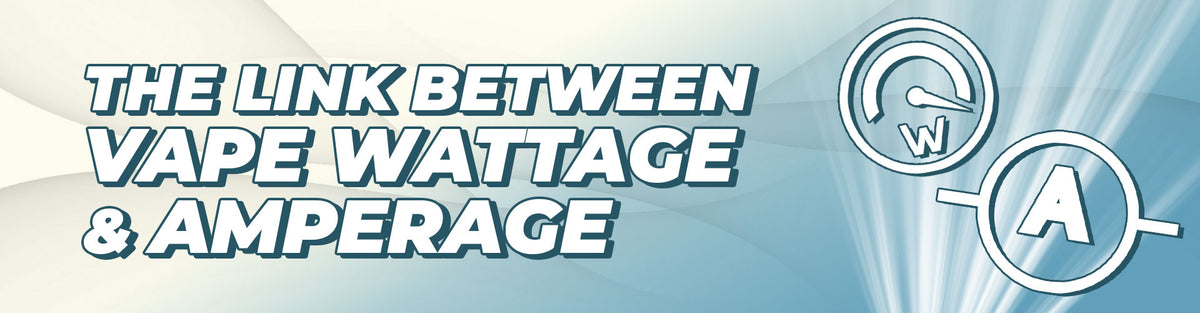#### The link between Vape Wattage and Amperage

If you’ve ever browsed our selection of vape kits, you’ve no doubt noticed that many of them include batteries with a capacity value given as numerical value and mAh. mAh is an abbreviation for milliamp hours, which is simply a smaller unit of amp hours. The reason for paying attention to the amp hour (Ah) value of your battery, is that it is tied to the wattage your device is operating at.

Specifically:

● An amp hour is simply a measure of how long a battery can provide one amp of power per hour.

● However, depending on what power settings you’re using on your vape kit, the amperage draw isn’t necessarily equal to 1A — it may be higher or lower.

● More typically, advanced vape kits allow you to directly adjust the output wattage of the electric circuit, rather than directly adjust the current flowing through it.

● As such, it may be helpful to be able to use two known values — output in W, battery life in Ah — to calculate more realistic battery runtimes.

A formula that we can use to calculate battery runtime is:

(10 x battery capacity in amp hours) divided by (appliance load in watts).

So let’s say you have a 3000mAh battery — which is the same as a 3Ah battery — and you’ve set your vape kit to deliver an output of 30W. You would then calculate as follows:

(10 x 3) ÷ 30 = 1

Note that while the answer is one hour in this example, this is by no means a worryingly low runtime. What this means is that the battery would be able to constantly output 30W for an entire hour before fully discharging. Of course, vape kits are not constantly delivering their maximum given output; this is simply their output level when firing or activated.

However, the able calculation can be handy in general. Although a given Ah or mAh value for your battery gives you a rough idea, by calculating Ah relative to your preferred wattage, you’ll get a slightly better sense of battery runtime.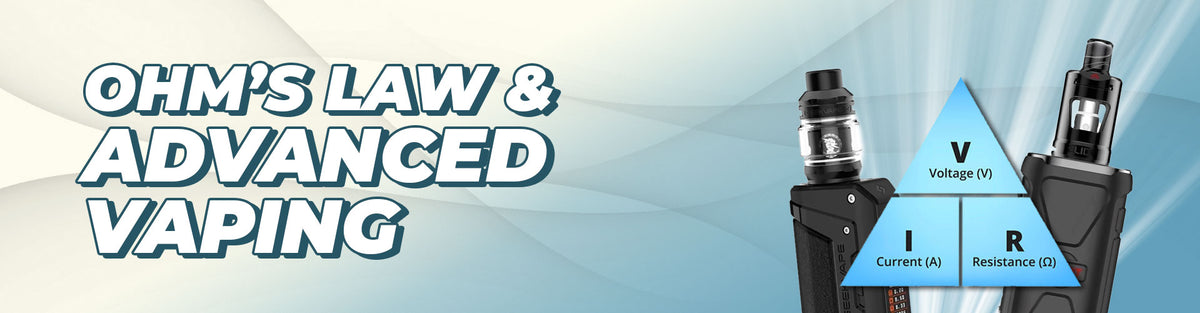### Ohm’s Law and Advanced Vaping

So, there you have it. As we’ve noted, Ohm’s law is really only applicable for particularly advanced vape users. That said, there are really only a few simple mathematical expressions to keep track, and you can potentially unlock high-level vape mastery. This will give you a much deeper understanding of why your vape kit works the way it does, and how to get the most out of your vaping experience.

At myCigara, we exist to make the UK smoke-free. We think that vaping is one of the best options out there for anyone looking to quit smoking for good, and that’s why we aim to deliver some of the industry’s leading vaping blogs. Our range of user guides have a focus on product education, aiming to give reader’s the know-how to make an informed decision about whether vaping is right for them.

If you’re totally new to vaping and accidentally landed on this page, we have some easier tips & guides available if you want to learn more. Alternatively, head into your nearest myCigara vape shop for a chat with our expert staff, or simply get in touch with us directly, and we’ll make sure you have the best possible first-time experience with vaping.

## You may also like to read....### How to Choose a Vape Kit

Feb 09, 2023 Jemma Wolfe### Water Based Vaping Explained

Jul 18, 2022 Jemma Wolfe### What is a Dry Hit?

Sep 26, 2022 Jemma Wolfe### Ultrasonic Vaping

Mar 24, 2022 Jemma Wolfe### Transitioning MTL to DTL Vaping

Oct 03, 2022 Theo Wilson